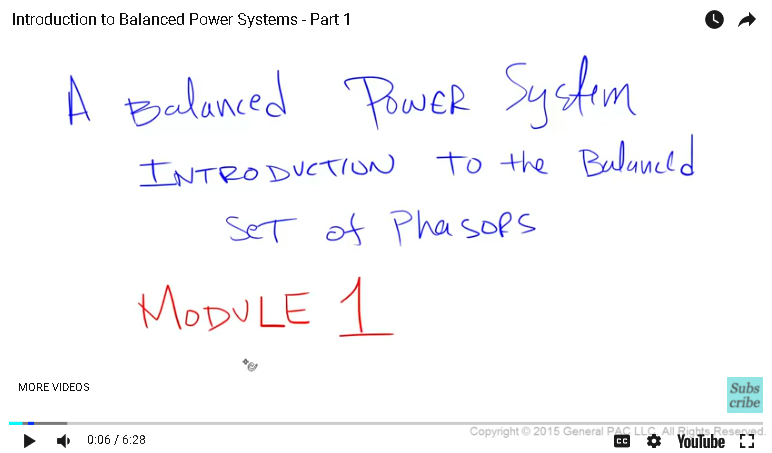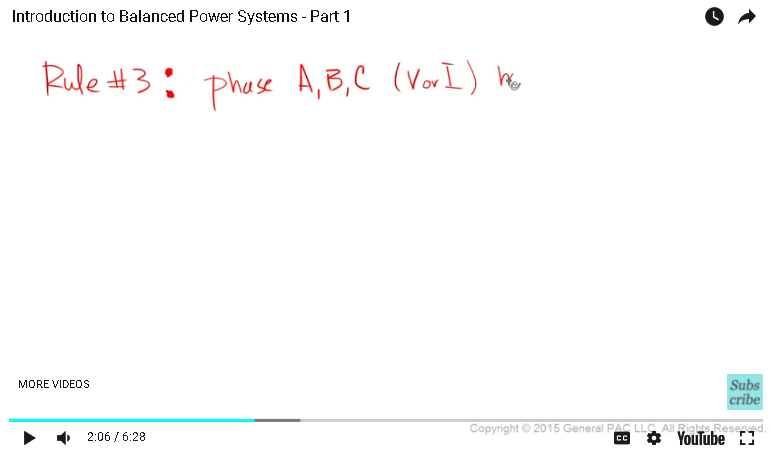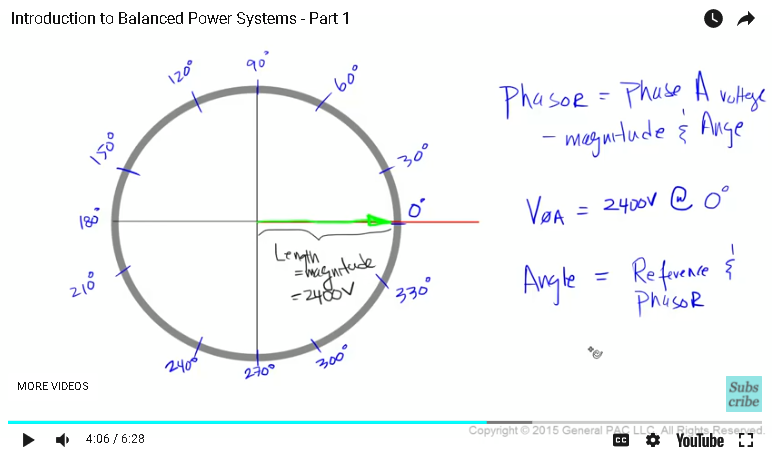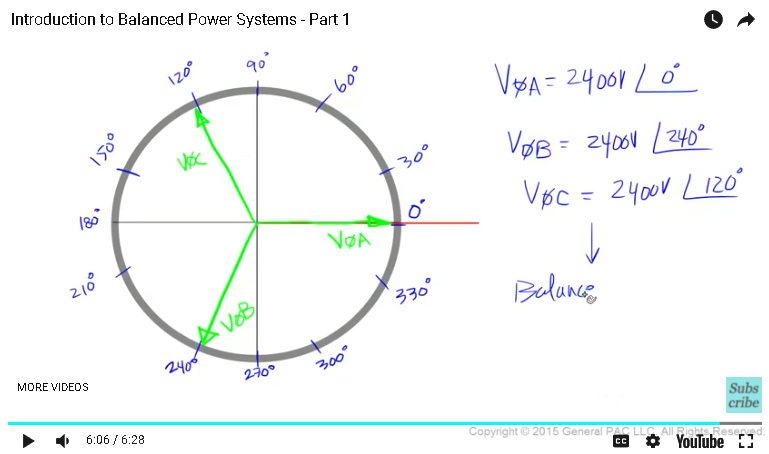This video was brought to you by GeneralPAC.com, making power systems Intuitive, Open and Free for Everyone, Everywhere. Consider subscribing and supporting through patreon.com/GeneralPAC. This is a mechanism for you to support us financially so we can continue making high quality power system video tutorials. Our corporate sponsor for this topic is AllumiaX.com from Seattle, Washington. Contact them for industrial and commercial power system studies.

A balanced power system, introduction to the balanced set of phasors. Module 1: Introduction to the balanced set of phasors.In this module, we are going to talk about the very important concept, called the “Balanced systems” or a balanced set of phasors, which is widely used in many areas of power system.

Be sure to check out the resources section under this video for helpful comments, suggestions and clarifications.

When thinking about transformers, transformer connections, generators, square root of 3, symmetrical components and many many other things about the power system.

We often assume that we are operating in a balanced condition or we have a balanced system or we are operating in a normal or a steady state condition or we have a balanced set of voltages or currents.

All these points employ one thing, “A Balanced System”. A Balanced System is simply a set of phasors and it could be voltages or currents that follow three rules at all times.

These 3 rules are;

Rule no 1: Phase A, B and C voltages or currents must have equal magnitude, or in other words, phase A voltage must equal to Phase B voltage and phase B voltage must equal to Phase C voltage. All 3 phasors must have equal magnitude value.

Rule no 2: Phase A, B and C voltages or currents must be equally displaced. Equal displacement between three phasors in a 360° circle implies a 120° phase displacement between all three phasors. This will become very clear with an example

Rule no 3: Phase A, B and C voltages or currents must have either an ABC or ACB phase rotation or phase sequence and must rotate in a counter clockwise direction.We will explain ABC or ACB phase rotation or phase sequence in just a little while, but the most important thing to remember about rule no 3 is that all 3 phasors must always rotate in a counter clockwise direction as per the IEEE and the ANSI standards. All 3 rules, contribute to something we called a “Balanced system”. So let’s illustrate the example and see if we follow all 3 rules.

We are going to draw a phasor diagram with an X-axis, Y-axis in nice round circle right in the middle. We are going to label degree marks at every 30° increments.

We will also draw a different colored line at the 0° mark. This is our reference line.

Okay, so first we will draw a phasor which will call phase A voltage, when thinking about the phasor, the magnitude and angle of that phasor should be very apparent.

So suppose, phase A voltage equals 2400 volts at 0°. We will represent phase A voltage by drawing a line starting at the origin and ending at the 0° mark.

So actual length of the phasor represents the magnitude of phase A voltage and we are assuming that the magnitude of phase A voltage equals 2400 volts,

So in other words, the length of a phasor represents 2400 volts. The angle of this phasor is represented by the angle between the reference line and the phasor.

Since the phasr overlaps the reference line or is in-phase with the reference line. We understand that there is a 0° between the phasor and the reference line.So, mathematically we will represent this phasor as a phase A voltage equals 2400 volts at 0°.

Next, we will draw another phasor and call it a phase B voltage, we will assume the phase B voltage equals 2400 volts at 240°.

We will illustrate phase B voltage by drawing a line from the origin towards a 240° mark. The magnitude of phase B voltage is 2400 volts which is represented by the length of thephasor. The angle of this phasor represented by the angle between the reference line and the phase B voltage.

So, begin at the reference line and rotate in a counter clockwise direction until we get to the phasor. The angle between the reference line and phasor is 240°.

Mathematically we will represent this phasor as a phase B voltage equals 2400 volts at 240°.

we will draw the last phasor and call it as a phase C voltage, we will assume that the phase C voltage equals 2400 volts at 120°.

So for phase C voltage, we are going to start at the origin, draw a line towards the 120° mark and stop here.

The magnitude of phase C voltage is 2400 volts and the angle between the reference line and the phase C voltage in the counter clockwise direction is a 120°

So, mathematically we will represent this phasor as phase C voltage equals 2400 volts at a 120°.

This my friend is what we call the balanced set of phasors, which contribute to something that we call a balanced system.In the next module, we will continue this example and make sure that we are following all 3 rules.

This module is brought to you buy GeneralPac.com. A platform to ask, learn, share and grow in a power system protection, automation and controls industry.

#### Greetings from the GeneralPAC Team!

We make high-quality Power Systems Video Tutorials on complex topics that are free and open to everyone!  Thank you so much for supporting us through Patreon so we can continue doing good and valuable work.

What is Patreon and why do we use it?

Patreon is a fantastic portal that allows our fans and community to make monthly contribution (like Netflix subscription) so we can continue creating high-quality power systems video tutorials. In return, you get access to incredible perks like voting on future topics, getting your questions answered, access to VIP Q/A webinars with the creators of GeneralPAC, and much more! We THANK YOU for supporting us

Why do we need your support?

An incredible amount of time and effort is needed to develop high-quality video tutorials. Each video (Part 1 for example) takes approximately 10 hours to complete which includes learning the concept ourselves, brainstorming creative ways to teach and explain the concepts, writing the script, audio recording, video recording, and editing. It's no wonder why Hundreds-of-Thousands of people have watched, liked, subscribed, and left positive comments on Youtube channel. Your support truly makes all the difference.